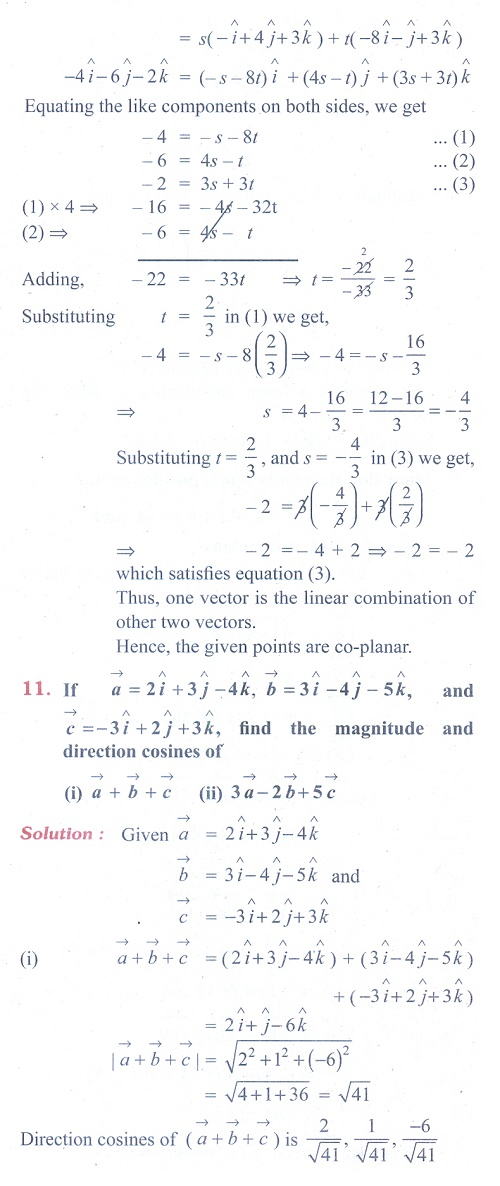Home | | Maths 11th std | Exercise 8.2: Direction Cosines and Direction Ratios

# Exercise 8.2: Direction Cosines and Direction Ratios

Maths Book back answers and solution for Exercise questions - Mathematics : Vector Algebra: Direction Cosines and Direction RatiosTags : Problem Questions with Answer, Solution | Mathematics , 11th Mathematics : UNIT 8 : Vector Algebra I
Study Material, Lecturing Notes, Assignment, Reference, Wiki description explanation, brief detail
11th Mathematics : UNIT 8 : Vector Algebra I : Exercise 8.2: Direction Cosines and Direction Ratios | Problem Questions with Answer, Solution | Mathematics